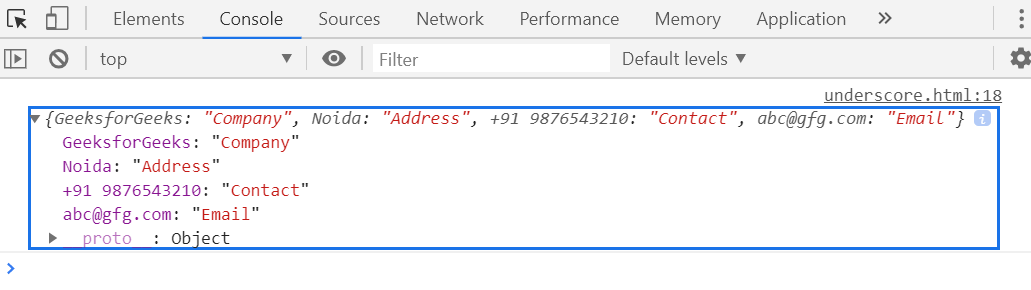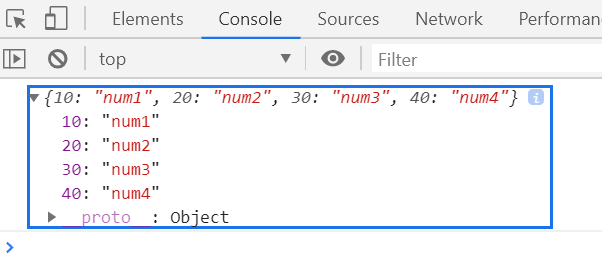# Underscore.js _.invert() Function

• Last Updated : 25 Nov, 2021

The _.invert() function is used to return the copy of an object where the object key is converted into value and object value is converted into the key. It means the [key, value] of the object is reverse.

Syntax:

`_.invert( object )`

Parameters: This function accepts single parameter as mentioned above and described below:

• object: It contains the object element that holds the elements of key and value pair.

Return Value: It returns the [key, value] of an object in reverse [value, key] order.

Example 1:

 ` ` `<``html``> ` ` `  `<``head``> ` `    ``<``script` `type``=``"text/javascript"`  `            ``src``= ` `"https://cdnjs.cloudflare.com/ajax/libs/underscore.js/1.9.1/underscore-min.js"``> ` `    `` ` ` ` ` `  `<``body``> ` `    ``<``script` `type``=``"text/javascript"``> ` ` `  `        ``var obj = { ` `            ``Company: "GeeksforGeeks", ` `            ``Address: "Noida", ` `            ``Contact: "+91 9876543210", ` `            ``Email: "abc@gfg.com" ` `        ``} ` `        ``console.log(_.invert(obj)); ` `    `` ` ` ` ` `  ` `

Output:Example 2:

 ` ` `<``html``> ` ` `  `<``head``> ` `    ``<``script` `type``=``"text/javascript"`  `            ``src``= ` `"https://cdnjs.cloudflare.com/ajax/libs/underscore.js/1.9.1/underscore-min.js"``> ` `    `` ` ` ` ` `  `<``body``> ` `    ``<``script` `type``=``"text/javascript"``> ` ` `  `        ``var inv = _.invert({ ` `            ``num1: 10, ` `            ``num2: 20, ` `            ``num3: 30, ` `            ``num4: 40 ` `        ``}); ` ` `  `        ``console.log(inv); ` `    `` ` ` ` ` `  ` `

Output:My Personal Notes arrow_drop_up
Recommended Articles
Page :